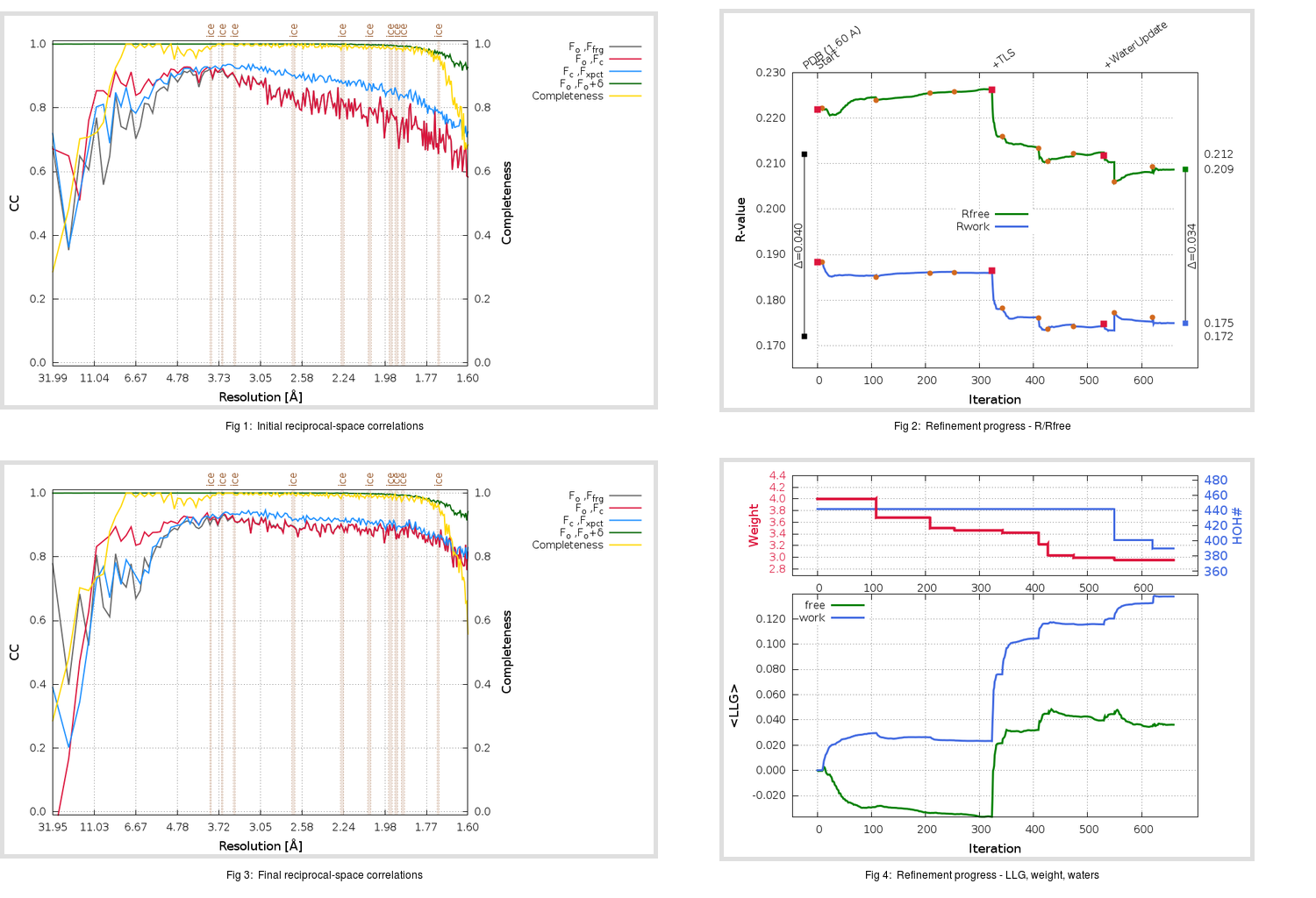Content:

## Deposited

` `
 Date deposited Date data collection Resolution R, Rfree 20130823 1.60 0.1700 0.2120

Molprobity (CCP4 7.0 version) summary:

```Ramachandran outliers =   0.00 %
favored =  98.34 %
Rotamer outliers      =   3.37 %
C-beta deviations     =     1
Clashscore            =   3.94
RMS(bonds)            =   0.0159
RMS(angles)           =   1.37
MolProbity score      =   1.58
Resolution            =   1.60
R-work                =   0.1700
R-free                =   0.2120
```

```Number of waters      =   442

<B> (all atoms) =   32.42 ( sd =   13.93 ) for       2868 non-hydrogen atoms
<B>   (protein) =   30.23 ( sd =   13.34 ) for       2380 non-hydrogen atoms
<B>     (water) =   44.57 ( sd =   10.53 ) for        442 non-hydrogen atoms
<B>    (others) =   27.38 ( sd =    9.39 ) for         46 non-hydrogen atoms

B min/max       (all non-hydrogen atoms) =   15.23 /  151.98
B min/max   (protein non-hydrogen atoms) =   15.23 /  151.98
B min/max     (water non-hydrogen atoms) =   18.34 /   71.96
B min/max     (other non-hydrogen atoms) =   19.64 /   49.89
```

## BUSTER (re-)refinement

` `

Molprobity (CCP4 7.0 version) summary:

```Ramachandran outliers =   0.66 %
favored =  97.01 %
Rotamer outliers      =   2.62 %
C-beta deviations     =     0
Clashscore            =   2.28
RMS(bonds)            =   0.0115
RMS(angles)           =   1.54
MolProbity score      =   1.50
Resolution            =   1.60
R-work                =   0.1749
R-free                =   0.2087
```

```Number of waters      =   390

<B> (all atoms) =   32.22 ( sd =   13.05 ) for       2816 non-hydrogen atoms
<B>   (protein) =   29.68 ( sd =    9.83 ) for       2380 non-hydrogen atoms
<B>     (water) =   48.00 ( sd =   18.02 ) for        390 non-hydrogen atoms
<B>    (others) =   27.96 ( sd =   10.95 ) for         46 non-hydrogen atoms

B min/max       (all non-hydrogen atoms) =   15.34 /  205.80
B min/max   (protein non-hydrogen atoms) =   15.34 /   71.72
B min/max     (water non-hydrogen atoms) =   19.13 /  205.80
B min/max     (other non-hydrogen atoms) =   19.99 /   52.71
```

Refinement progression:Results:

` `
 File Remark 4MDS_aB_refine.01_03_refine.pdb.gz exact refinement commands are in header 4MDS_aB_refine.01_03_refine.mtz.gz including original deposited data and several re-refinement map coefficients 4MDS_aB_refine.01_03_BUSTER_model.cif.gz including any non-standard compound restraints 4MDS_aB_refine.01_03_BUSTER_refln.cif.gz